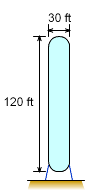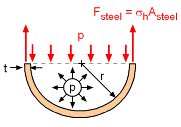Ch 2. Fluid Statics Multimedia Engineering Fluids PressureVariation PressureMeasurement HydrostaticForce(Plane) HydrostaticForce(Curved) Buoyancy
 Chapter 1. Basics 2. Fluid Statics 3. Kinematics 4. Laws (Integral) 5. Laws (Diff.) 6. Modeling/Similitude 7. Inviscid 8. Viscous 9. External Flow 10. Open-Channel Appendix Basic Math Units Basic Equations Water/Air Tables Sections Search eBooks Dynamics Fluids Math Mechanics Statics Thermodynamics Author(s): Chean Chin Ngo Kurt Gramoll ©Kurt GramollFLUID MECHANICS - EXAMPLE QuestionsWater StandpipeFree Body Diagram One type of water tank for a municipal water supply system is a standpipe. If the standpipe is 120 ft tall and the diameter is 30 ft, calculate the stress in the 0.5 in. thick steel plates at the bottom of the tank. Assume both ends are spherical and the tank is completely filled with water. Ignore minor pressure variations in curved ends. Solution The dimensions of the standpipe are:      120 ft tall      d = 30 ft or r = 15 ft      t = 0.5 in Water pressure (hydrostatic pressure variation) at the bottom of the standpipe     p = γw z = (62.4 pcf)(120 ft)        = 7,488 psf = 52.0 psi From the free body diagram, for equilibrium Fs = pA For unit thickness (pressure variation negligible in the limit): σh (2r π) (t) = p (πr2) The hoop stress is calculated as      σh = pr / 2t = (52 psi)(15 ft)(12 in/ft) / [2 (0.5 in)]          = 9,360 psi = 9.36 ksi Note 1: the tensile strength of steel is on the order of 40 - 80 ksi, so 0.5 in. is sufficient thickness. Note 2: final plate thickness used in design must consider wind and/or earthquake loads.

Practice Homework and Test problems now available in the 'Eng Fluids' mobile app
Includes over 250 problems with complete detailed solutions.
Available now at the Google Play Store and Apple App Store.